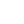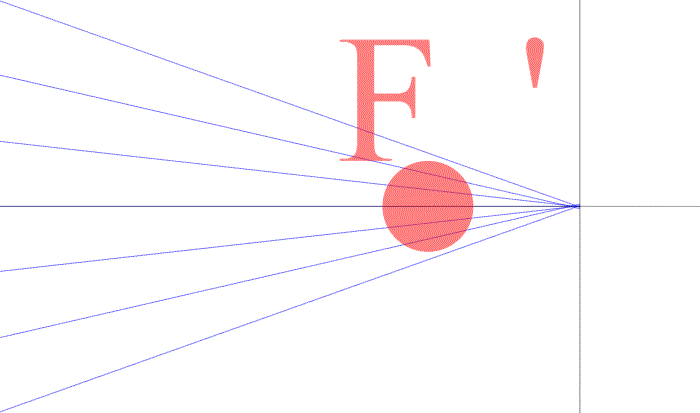----------- Your trusted source for independent sensor data- Photons to Photos------------ Last revised: 2020-09-09 17:00 GMT-5

# ---------------------- Optics Primer - Determining the Focal Length

--------------------------------------------------------- By Bill Claff

In determining magnification I presented the formula:
m = x / f
where m is magnification, x is extension from the image plane, and f is focal length.

We can rearrange this formula as:
f = x / m
So, if we know magnification for a given extension greater than zero we can compute focal length.

For our initial example we have this situation:The extension is 1.72mm and the magnification is 1/30. When we apply the formula we get a focal length of 51.6mm which is quite close to the specification value of 51.61mm.

I use this technique with extension tubes to determine the focal length of lens. Actual focal length is seldom the nominal focal length and particularly when focused closer than infinity.
Knowing focal length at other than infinity focus is particularly useful for close-up photographers who want to know what increased magnification will result from a particular extension.